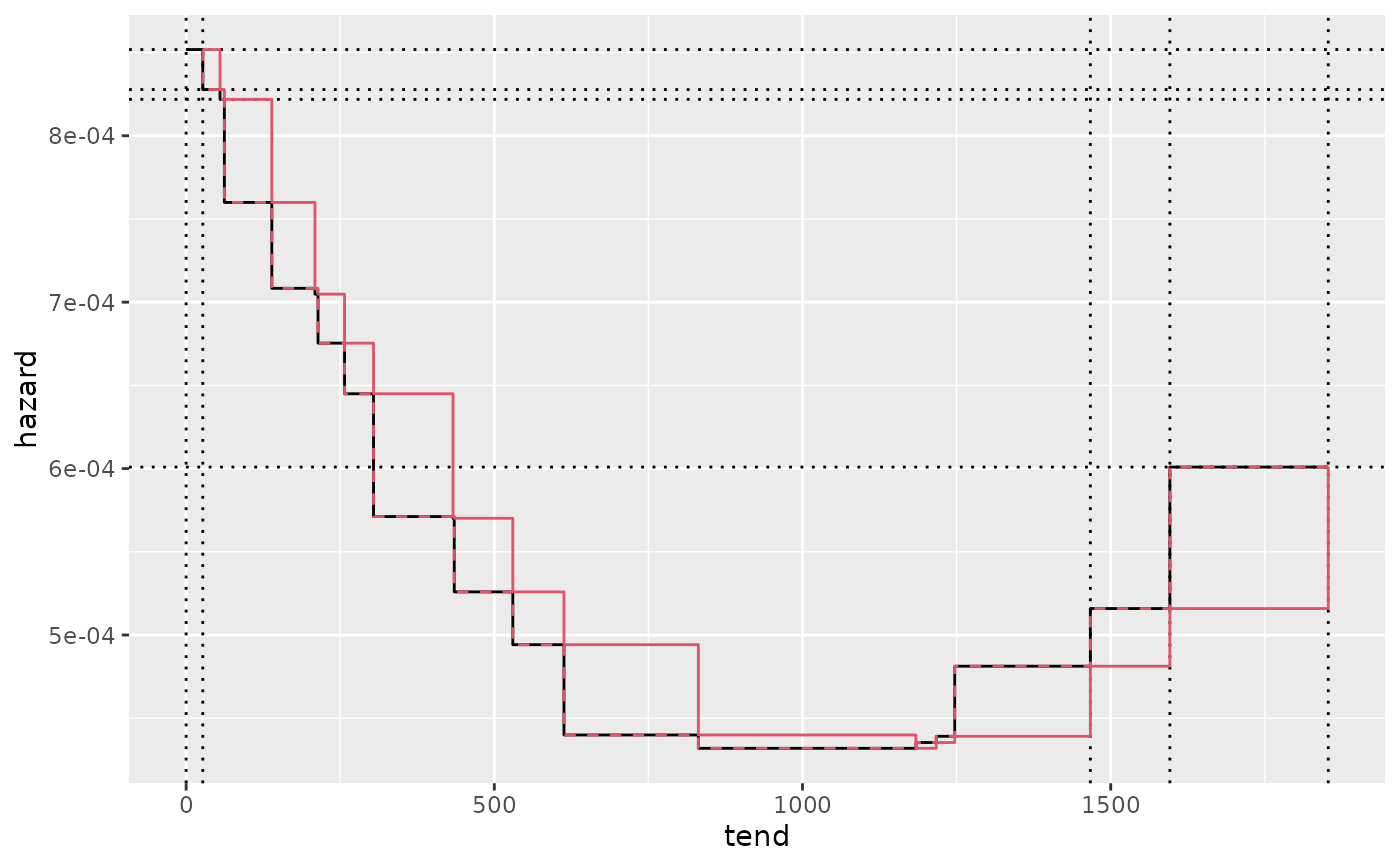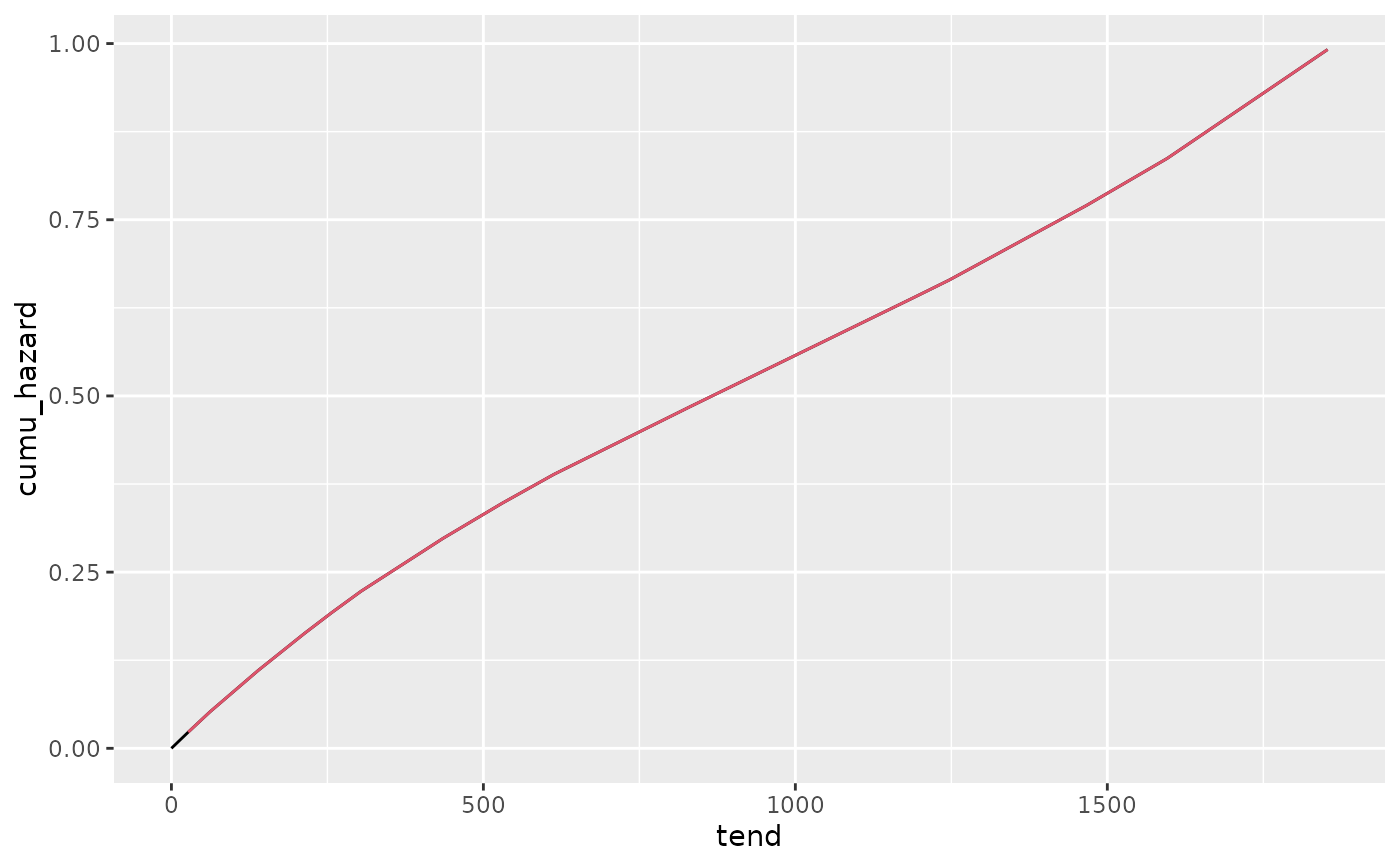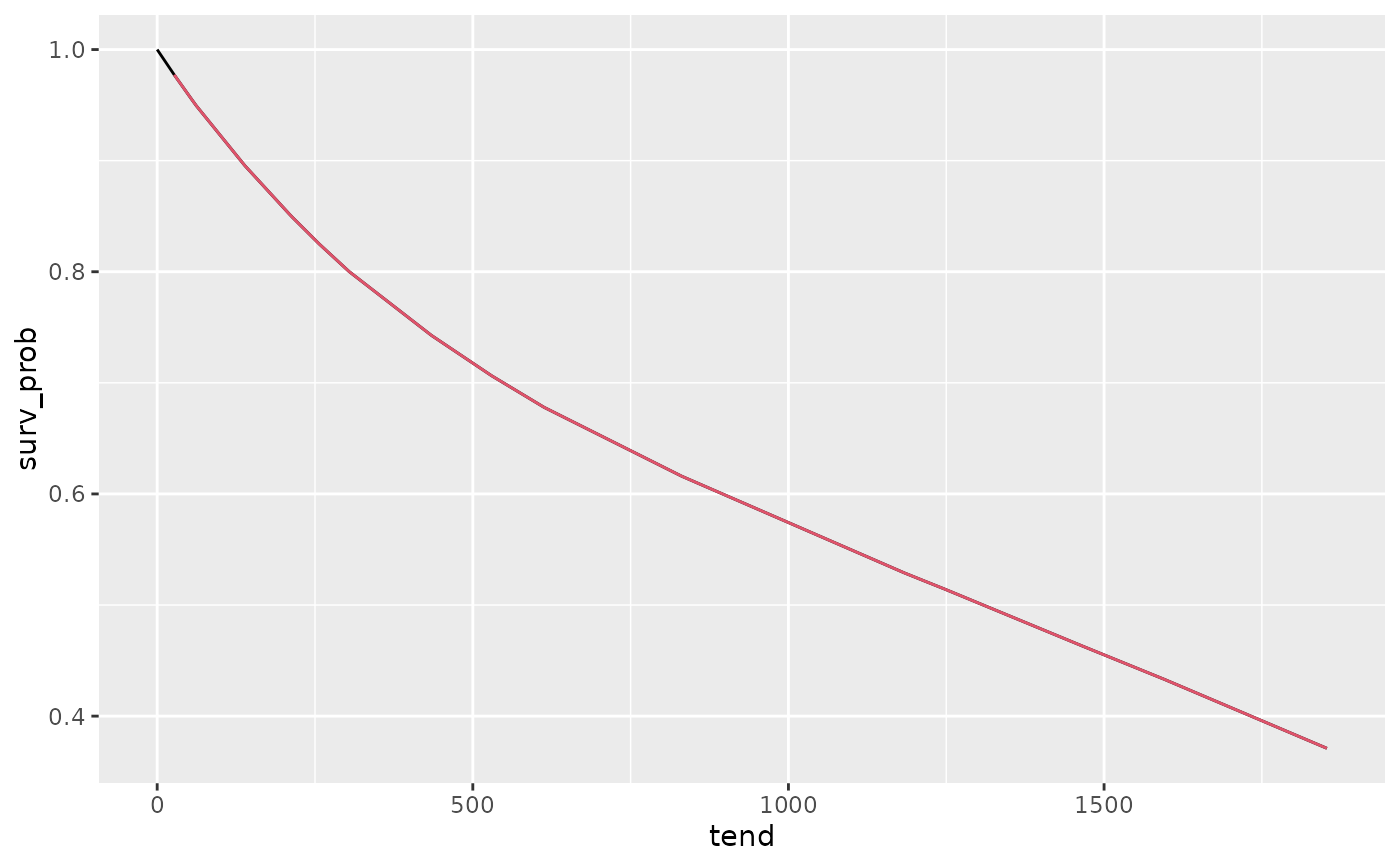geom_hazard is an extension of the geom_line, and is optimized for (cumulative) hazard plots. Essentially, it adds a (0,0) row to the data, if not already the case. Stolen from the RmcdrPlugin.KMggplot2 (slightly modified).

geom_hazard(
mapping = NULL,
data = NULL,
stat = "identity",
position = "identity",
na.rm = FALSE,
show.legend = NA,
inherit.aes = TRUE,
...
)

geom_stephazard(
mapping = NULL,
data = NULL,
stat = "identity",
position = "identity",
direction = "vh",
na.rm = FALSE,
show.legend = NA,
inherit.aes = TRUE,
...
)

geom_surv(
mapping = NULL,
data = NULL,
stat = "identity",
position = "identity",
na.rm = FALSE,
show.legend = NA,
inherit.aes = TRUE,
...
)

## Arguments

mapping

Set of aesthetic mappings created by aes(). If specified and inherit.aes = TRUE (the default), it is combined with the default mapping at the top level of the plot. You must supply mapping if there is no plot mapping.

data

The data to be displayed in this layer. There are three options:

If NULL, the default, the data is inherited from the plot data as specified in the call to ggplot().

A data.frame, or other object, will override the plot data. All objects will be fortified to produce a data frame. See fortify() for which variables will be created.

A function will be called with a single argument, the plot data. The return value must be a data.frame, and will be used as the layer data. A function can be created from a formula (e.g. ~ head(.x, 10)).

stat

The statistical transformation to use on the data for this layer, either as a ggproto Geom subclass or as a string naming the stat stripped of the stat_ prefix (e.g. "count" rather than "stat_count")

position

Position adjustment, either as a string naming the adjustment (e.g. "jitter" to use position_jitter), or the result of a call to a position adjustment function. Use the latter if you need to change the settings of the adjustment.

na.rm

If FALSE, the default, missing values are removed with a warning. If TRUE, missing values are silently removed.

show.legend

logical. Should this layer be included in the legends? NA, the default, includes if any aesthetics are mapped. FALSE never includes, and TRUE always includes. It can also be a named logical vector to finely select the aesthetics to display.

inherit.aes

If FALSE, overrides the default aesthetics, rather than combining with them. This is most useful for helper functions that define both data and aesthetics and shouldn't inherit behaviour from the default plot specification, e.g. borders().

...

Other arguments passed on to layer(). These are often aesthetics, used to set an aesthetic to a fixed value, like colour = "red" or size = 3. They may also be parameters to the paired geom/stat.

direction

direction of stairs: 'vh' for vertical then horizontal, 'hv' for horizontal then vertical, or 'mid' for step half-way between adjacent x-values.

geom_line, geom_step.

## Examples

library(ggplot2)
library(pammtools)
ped <- tumor[10:50,] %>% as_ped(Surv(days, status)~1)
pam <- mgcv::gam(ped_status ~ s(tend), data=ped, family = poisson(), offset = offset)
ndf <- make_newdata(ped, tend = unique(tend)) %>% add_hazard(pam)
# piece-wise constant hazards
ggplot(ndf, aes(x = tend, y = hazard)) +
geom_vline(xintercept = c(0, ndf$tend[c(1, (nrow(ndf)-2):nrow(ndf))]), lty = 3) + geom_hline(yintercept = c(ndf$hazard[1:3], ndf\$hazard[nrow(ndf)]), lty = 3) +
geom_stephazard() +
geom_step(col=2) +
geom_step(col=2, lty = 2, direction="vh")
#> Warning: Unknown or uninitialised column: linewidth.
#> Warning: Unknown or uninitialised column: size.# comulative hazard
ggplot(ndf, aes(x = tend, y = cumu_hazard)) +
geom_hazard() +
geom_line(col=2) # doesn't start at (0, 0)
#> Warning: Unknown or uninitialised column: linewidth.
#> Warning: Unknown or uninitialised column: size.# survival probability
#> Warning: Unknown or uninitialised column: linewidth.
#> Warning: Unknown or uninitialised column: size.